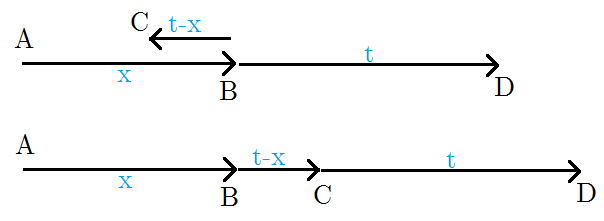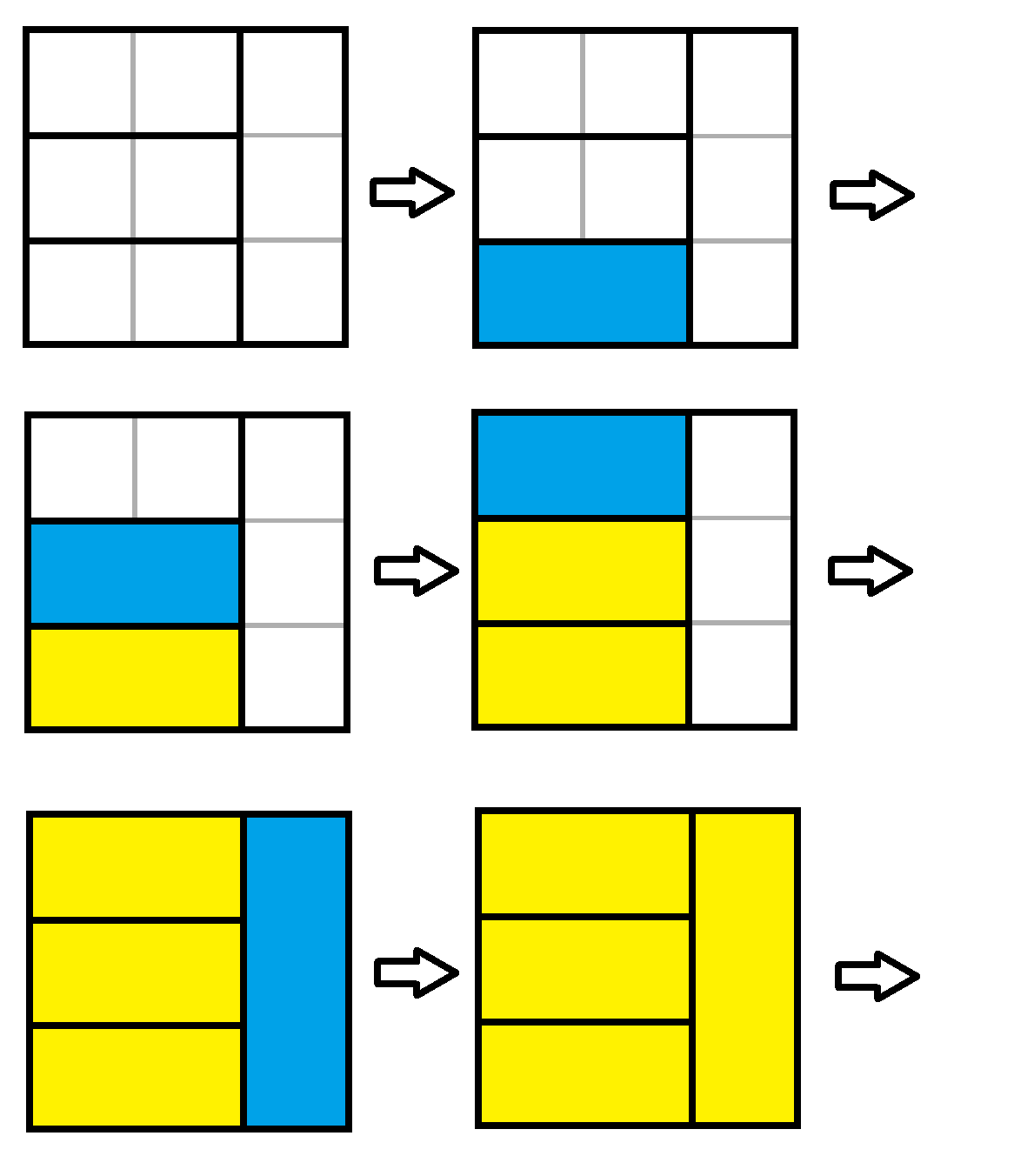SocialZxy's Blog 2021-01-10T04:24:16.399Z http://socialzxy.github.io/ SocialZxy Hexo 贪心口胡 http://socialzxy.github.io/2021/01/06/%E8%B4%AA%E5%BF%83%E5%8F%A3%E8%83%A1/ 2021-01-06T03:45:14.000Z 2021-01-10T04:24:16.399Z 前言

## POJ1700 Crossing River

1. $a$ 送 $b$ 过河，$a$ 返回，$c$ 送 $d$ 过河，$b$ 返回，贡献为 $a+2b+d$
2. $a$ 送 $c$ 过河，$a$ 返回，$a$ 送 $d$ 过河，$a$ 返回，贡献为 $2a+c+d$

## P3457 [POI2007]POW-The Flood

[CENSORED]：赵队不知道自己在写什么。

[CENSORED]：啊这，这不是有手就行吗？￥*&……#￥

## P4437 [HNOI-AHOI2018]排列

1. 它的父亲现在已经填入序列中了。我们可以直接将其放在最前面。
2. 它的父亲现在还没填入，那么我们应该让它填在父亲后面恰好一位，相当于将父亲和它粘在一起。

$$\Delta sum_1=\sum_{i=1}^{cnt1}(now+i)w_i+\sum_{i=1}^{cnt2}(now+cnt1+i)w'_i$$$$\Delta sum_2=\sum_{i=1}^{cnt2}(now+i)w^\prime_i+\sum_{i=1}^{cnt1}(now+cnt2+i)w_j$$

## UOJ387. 【UNR #3】To Do Tree

[CENSORED]：这个结论不是很显然吗？

## LOJ2392. 「JOISC 2017 Day 1」烟花棒

### 证明

1. 马上点燃 B，然后两个人同向跑。
2. 马上点燃 B，然后两个人反向跑。
3. B 先陪跑，等 A 快没了的时候再点燃 B。## 注释

「迫害」栏目的所有 [CENSORED] 都是同一个人

]]>

## Improvement

• 找到 $[l,r]$ 的中点 $mid$，将所有小于 $mid$ 的数扔进树状数组。
• 统计每个询问的答案，如果不小于 $k$ 扔到左边，否则将 $k$ 减去这个答案然后扔到右边。
• 递归下去处理左边 $solve(l, mid, x, div)$ 和右边 $solve(mid + 1, r, div+1, y)$。
• 当 $l=r$ 时，结束递归，确定当前所有考虑的询问的答案。
• 记得用正确的方法清空树状数组

## Example

P3242 [HNOI2015] 接水果

1. 首先对于询问的格式，我们是将所有信息（初始信息、查询区间）全都揉进一个数组里面，分别标上 $0,1$ 的标签。显然需要先修改后查询，所以在调用函数前需要先排个序，让 0 在 1 的前面（当然扫描线要求 $x$ 递增，这个也要考虑进去）
2. 如果当前没有需要考虑的询问了，直接返回。
3. 如果二分答案区间相等说明找到答案了，更新此时考虑的所有询问的答案。
4. 接下来需要做扫描线，这样讨论一下：
1. 如果当前询问标签为 $0$，说明这是个修改类型的询问，如果其 $k$ 值小于 $mid$ 那么把它扔进树状数组，并放进 $q1$ 中临时存储。否则不修改树状数组，并将这个询问扔进 $q2$ 中存储。
2. 如果当前询问标签为 $1$，说明这是个查询类型的询问，在树状数组上面单点查询得到覆盖此点的矩形数量，如果 $k$ 不大于这个数量，那么将其放进 $q1$ 中临时存储，否则将其放入 $q2$ 中临时存储。
5. 将 $q1$ 和 $q2$ 按顺序排回去，由于我们做的是一个稳定的筛选过程，所以这样排回去依然是满足扫描线扫描顺序的。
6. 递归向下处理。

]]>

## P2303 [SDOI2012] Longge 的问题

$$\sum_{d|n}d\sum_{i=1}^n[(d,i)=d]=\sum_{d|n}d\sum_{i=1}^{n/d}[(d,i)=1]=\sum_{d|n}d\varphi(n/d)$$

## P2257 YY的GCD

$$\sum_{i=1}^n\sum_{j=1}^m[gcd(i,j)\in P]$$$$=\sum_{p\in P}\sum_{i=1}^n\sum_{j=1}^m[gcd(i,j)=p]$$$$=\sum_{p\in P}\sum_{i=1}^{n/p}\sum_{j=1}^{m/p}[gcd(i,j)=1]$$$$=\sum_{p\in P}\sum_{i=1}^{n/p}\sum_{j=1}^{m/p}\sum_{d|i,d|j}\mu(d)$$$$=\sum_{p\in P}\sum_{d=1}^{n/p}\mu(d)\lfloor n/dp\rfloor\lfloor m/dp\rfloor$$

$$\sum_{T=1}^n\lfloor n/T\rfloor\lfloor m/T\rfloor\sum_{p\in P|T}\mu(T/p)$$

## P3327 [SDOI2015]约数个数和

### Lemma

$$d(ij)=\sum_{x|i}\sum_{y|j}[x\perp y]$$

### 证明（口胡）

• 在 $i$ 中选择，需要满足情况 $c\leq a$，$p^c=p^\alpha(\alpha\leq a)$。
• 在 $j$ 中选择，需要满足情况 $c>a$，$p^c=p^a\times p^\beta(\beta \leq b)$。

$$sum_{i=1}^n\sum_{j=1}^md(ij)$$$$=\sum_{i=1}^n\sum_{j=1}^m\sum_{x|i}\sum_{y|j}[(x,y)=1]$$$$=\sum_{i=1}^n\sum_{j=1}^m\sum_{x|i}\sum_{y|j}\sum_{d|x,d|y}\mu(d)$$$$=\sum_{x=1}^n\sum_{y=1}^m\lfloor n/x\rfloor\lfloor m/y\rfloor\sum_{d|x,d|y}\mu(d)$$$$=\sum_{d=1}^n\mu(d)\sum_{d|x}^n\sum_{d|y}^m\lfloor n/x\rfloor\lfloor m/y\rfloor$$$$=\sum\mu(d)\sum_{x=1}^{n/d}\sum_{y=1}^{m/d}\lfloor n/xd\rfloor\lfloor m/yd\rfloor$$$$=\sum\mu(d)(\sum_{x=1}^{n/d}\lfloor n/dx\rfloor)(\sum_{y=1}^{m/d}\lfloor m/dy\rfloor)$$

## SP4168 SQFREE - Square-free integers

$$n+\sum_{i=2}^{\sqrt n} \mathrm{sign}(i) \lfloor n/i^2\rfloor$$

• 若 $i$ 本身有平方因子，那么 $i$ 的倍数的贡献无需在此计算，$\mathrm{sign}(i)=0$；
• 若 $i$ 本身没有平方因子，那么：
• 只有一个素因子的应该减去，$\mathrm{sign}(x)=-1$；
• 只有两个素因子的在只有一个素因子的部分已经计算过了两次，应该加回来，$\mathrm{sign}(x)=1$；
• 只有三个素因子的在前面被多加了一次，应该再减去， $\mathrm{sign}(x)=1$；
• 以此类推。

## P3312 [SDOI2014]数表

$$sum_{i=1}^n\sum_{j=1}^m\sigma_0(\gcd(i,j))$$$$=\sum_{p=1}^n\sigma_0(p)\sum_{i=1}^n\sum_{j=1}^m[\gcd(i,j)=p]$$$$=\sum_{p=1}^n\sigma_0(p)\sum_{i=1}^{n/p}\sum_{j=1}^{m/p}\sum_{d|i,d|j}\mu(d)$$$$=\sum_{p=1}^n\sigma_0(p)\sum_{d=1}^{n/d}\mu(d)\lfloor n/dp\rfloor\lfloor m/dp\rfloor$$$$=\sum_{T=1}^n\lfloor n/T\rfloor\lfloor m/T\rfloor\sum_{d|T}\mu(T/d)\sigma_0(d)$$

## P1587 [NOI2016]循环之美

### Lemma

$$\sum_{i=1}^n\sum_{j=1}^m[i\perp j][j\perp k]$$

### 证明

$$sum_{i=1}^n\sum_{j=1}^m[i\perp j][j\perp k]$$$$=\sum_{j=1}^m[j\perp k]\sum_{i=1}^n\sum_{d|i,d|j}\mu(d)$$$$=\sum_{d=1}^n\mu(d)\sum_{d|i}\sum_{d|j}[j\perp k]$$$$=\sum_{d=1}^n\mu(d)[d\perp k]\lfloor n/d\rfloor\sum_{j=1}^{m/d}[j\perp k]$$

$$F(x)=\sum_{i=1}^x[i\perp k]$$

$$S(x)=\sum_{i=1}^x\mu(i)[i\perp k]$$

$$F(x)=\lfloor x/k \rfloor f(k)+f(x\bmod k)$$

$$S(x)=\sum_{i=1}^x\mu(i)[d\perp k]$$$$=\sum_{i=1}^x\mu(i)\sum_{d|i,d|k}\mu(d)$$$$=\sum_{d|k}\mu(d)\sum_{i=1}^{x/d}\mu(id)$$

$$S(x)=\sum_{d|k}\mu(d)\sum_{i=1}^{x/d}[i\perp d]\mu(i)\mu(d)$$$$=\sum_{d|k}\mu^2(d)\sum_{i=1}^{x/d}[i\perp d]\mu(i)$$

$$S(x,y)=\sum_{i=1}^x\mu(i)[i\perp y]$$

$$S(x,y)=\sum_{d|y}\mu^2(d)S(x/d,d)$$

## P4619 [SDOI2018]旧试题

]]>

2020. 12. 02 水题 http://socialzxy.github.io/2020/12/02/20201202%E6%B0%B4%E9%A2%98/ 2020-12-02T03:45:14.000Z 2020-12-04T07:13:42.223Z 2020. 12. 02 水题

## P2507 [SCOI2008]配对

]]>
2020. 12. 02 水题
2020. 11. 30 & 12. 01 水题 http://socialzxy.github.io/2020/12/01/20201201%E6%B0%B4%E9%A2%98/ 2020-12-01T03:45:14.000Z 2020-12-04T07:13:13.765Z 2020. 11. 30 & 12. 01 水题

## MZOJ #380. 情报传输

• $f[i][j]$ ： $i$ 的 $2^j$ 祖先。
• $g[i][j]$ ：从 $i$ 向上拉出去并且不再拉回本子树，向上最远走到 $f[i][j]$ 的最长链。
• $h[i][j]$ ：从 $f[i][j]$ 往下走，不走进 $i$ 的子树中的最长链。

1. $rt$ 是 $x$ 的祖先，那么统计 $x$ 子树中的最长链，再把 $x$ 往上跳，同时统计 $g$
2. $x$ 是 $rt$ 的祖先，那么从 $yfa$向上跳到 $x$，统计 $h$，把 $x$ 向上跳到 $1$，统计 $g$
3. $x$ 和 $rt$ 不在同一子树中，那么分成几部分：首先将 $x$ 向上跳到 $lca$，同时统计 $g$，将 $yfa$ 向上跳到 $lca$，同时统计 $h$，然后在 $lca$ 处检查最长链、次长链、第三长链，然后从 $lca$ 跳到 $1$，统计 $g$

## MZOJ #381. 旅游路线

]]>
2020. 11. 30 & 12. 01 水题
2020. 11. 24 & 25 水题 http://socialzxy.github.io/2020/11/25/20201124%E6%B0%B4%E9%A2%98/ 2020-11-25T03:45:14.000Z 2020-12-04T07:12:28.888Z 2020. 11. 24 & 25 水题

## GMOJ 6887. 路径

（对不起今晚真的没时间了，以后有时间再来补具体的吧）

## GMOJ 6890. 打字机

• 压一个字符
• 弹出栈顶
• 打印栈顶

$XYZ$ 只有 $6$ 种可能，分类讨论一下随便做一个 $DP$ 就可以了。

（好像有点糊，不过不管了）

## GMOJ 6892. 树的解构

$$f_i=\sum_{j=1}^i(j-1)!(f_{i-j}+(i-j)!)\binom{i-1}{i-j}$$

$$f_i=(i-1)!\sum_{j=1}^i\dfrac{f_{i-j}}{(i-j)!}+i(i-1)!$$

## GMOJ 6891. 太空漫步

]]>
2020. 11. 24 & 25 水题
2020. 11. 23 水题 http://socialzxy.github.io/2020/11/23/20201123%E6%B0%B4%E9%A2%98/ 2020-11-23T03:45:14.000Z 2021-01-10T03:44:46.278Z 2020. 11. 23 水题

## GMOJ 6881. T3 是我的你不要抢

• 长度大于 $\sqrt{L}$ 的串最多有 $\sqrt{L}$ 个。
• 求解两个串答案的时间复杂度为 $min(|S|, |T|)$。

## CF1214F Employment

1. $x-y$，应满足 $x\geq y, x-y\leq m/2$，贡献为 $x$。

2. $m-x+y$，应满足 $x\geq y, x-y\geq m/2$，贡献为 $-x$

3. $y-x$，应满足 $x\leq y, y-x< m/2$，贡献为 $-x$。

4. $m-y+x$，应满足 $x\leq y, y-x > m/2$，贡献为 $x$

我们可以先对于每一个 $a$，求出它产生每一类贡献的范围，区间加，然后对 $b$ 也做一遍类似的过程。

]]>
2020. 11. 23 水题

## 基本思想• 任意一种方案都可以由这种方法转移得出。
• 已经处理部分（有色部分）和未处理部分（无色部分）的分界线只会向下或向右延申。

$$(110010)_2$$

$$(100110)_2$$

• 预处理出所有的分界线并且按已处理部分面积排序。
• 找到每个状态中分界线上的下凸点，通过二进制反转的方式来转移。
• 最后 $F_{(00\cdots011\cdots1)_2}$ 就是答案。

## 习题

1. （矩阵取数）给定一个矩阵，你每次可以做这两种操作之一：

• 拿走一个数，代价为这个数
• 拿走两个数，要求这两个数右下方都没有其他数，代价为这两个数之和的绝对值

求最小的代价之和

2. （矩阵涂色）给定一个矩阵，你需要在上面涂上恰好 $k$ 种颜色，满足任意一条从左上角到右下角且只能向右或向下的路径上不出现两种同样的颜色，求方案数。

]]>

2020. 11. 22 水题 http://socialzxy.github.io/2020/11/22/20201122%E6%B0%B4%E9%A2%98/ 2020-11-22T03:45:14.000Z 2020-12-04T07:11:56.660Z 2020. 11. 22 水题

## GMOJ 6879. T1 出了个大阴间题

$$a’=max(a_1,a_2)+[a_1=a_2]\ b’=2max(b_1,b_2)+1$$

## CF1437E Make It Increasing

$$a_1+1\leq a_2\ a_2+1\leq a_3\ a_3+1\leq a_4\ \cdots$$

$$a_1\leq a_2-1\ a_1\leq a_3-2\ a_1\leq a_4-3\ \cdots$$

]]>
2020. 11. 22 水题
2020. 11. 20 水题 http://socialzxy.github.io/2020/11/20/20201120%E6%B0%B4%E9%A2%98/ 2020-11-20T03:45:14.000Z 2020-11-22T08:40:52.321Z 2020. 11. 20 水题

## GMOJ 6875. 背包

• 将一个物品加入背包，直接暴力 $DP$。
• 求两个背包合并后重量不超过 $m$ 的最大价值，求出一个背包的前缀最大值然后和后面的背包拼起来即可。

• ZXY：这个怎么 $O(m)$ 做啊？
• WTY：两个指针扫啊
• ZXY：你讲详细点
• WTY：详细点，就是两个指针扫啊

## GMOJ 6876. 祖先

]]>
2020. 11. 20 水题
2020. 11. 29 水题 http://socialzxy.github.io/2020/11/20/20201129%E6%B0%B4%E9%A2%98/ 2020-11-20T03:45:14.000Z 2020-12-04T07:12:47.611Z 2020. 11. 29 水题

## CF1437F Emotional Fishermen

$$H_1S\cdots SH_2S\cdots SH_3S\cdots$$

$$f_i=\sum_{j=0}^{i-1}f_j\times A(n-lim_j-2,lim_i-lim_j-1)$$

## CF1451D Circle Game

• 必胜态和必败态可以连成若干斜率为 $1$ 的直线，其中必胜态连成的线称为必胜线，反之称为必败线。
• 必胜线和必败线交替出现。

## P3745 [六省联考2017]期末考试

]]>
2020. 11. 29 水题
2020. 11. 19 水题 http://socialzxy.github.io/2020/11/19/20201119%E6%B0%B4%E9%A2%98/ 2020-11-19T03:45:14.000Z 2020-11-22T08:40:12.278Z 2020. 11. 19 水题

## CF802L Send the Fool Further! (hard)

$$\begin{cases} f_u=\dfrac{1}{deg_u}[\sum\limits_{v\in son_u}(f_v+w_{u,v})+f_{fa}+w_{fa,u}], u \notin Leaf\ f_u=0, u\in Leaf \end{cases}$$

\begin{aligned} deg_uf_u&=\sum_{v\in son_u}(A_vf_u+B_v)+f_{fa}+\sum_{u,v\in E}w_{u,v}\ (deg_u-\sum_{v\in son_u}A_v)f_u&=f_{fa}+(\sum_{v\in son_u}B_v+\sum_{u,v\in E}w_{u,v})\ f_u&=\dfrac{1}{deg_u-\sum_{v\in son_u}A_v}f_{fa}+\dfrac{\sum_{v\in son_u}B_v+\sum_{u,v\in E}w_{u,v}}{deg_u-\sum_{v\in son_u}A_v} \end{aligned}

$$\begin{cases} A_u=\dfrac{1}{deg_u-\sum_{v\in son_u}A_v},B_u=\dfrac{\sum_{v\in son_u}B_v+\sum_{u,v\in E}w_{u,v}}{deg_u-\sum_{v\in son_u}A_v},u\notin Leaf\ A_u=B_u=0, u\in Leaf \end{cases}$$

## GMOJ 6873. 飞翔的鸟

$$X’=XA, Y’=XB+YA$$

$$\begin{bmatrix}X\Y\end{bmatrix}\times \begin{bmatrix}A~~0\B~A\end{bmatrix}=\begin{bmatrix}X’\Y’\end{bmatrix}$$

## GMOJ 6874. ZYT的答案

]]>
2020. 11. 19 水题
2020. 11. 17 & 18 水题 http://socialzxy.github.io/2020/11/18/20201117%E6%B0%B4%E9%A2%98/ 2020-11-18T03:45:14.000Z 2020-11-22T08:39:27.662Z 2020. 11. 17 & 18 水题

## 洛谷 P5280 [ZJOI2019]线段树

• 与修改区间相交。
• 本身被包含，父亲不被包含。
• 本身相离，父亲相交。
• 本身被包含，父亲也被包含
• 完全无关

• 第一类，有一半的 LazyTag 没有了，变为 $(f_u/2, g_u/2)$。
• 第二类，有一半的 LazyTag 变成了 $1$，变为 $(f_u/2+1/2, g_u/2+1/2)$。
• 第三类，会被父亲 pushdown，变为 $(f_u/2+g_u/2, g_u)$。
• 第四类，LazyTag 不变，但是有一半到根路径上的 LazyTag 数量变成了 1，变为 $(f_u, g_u/2 + 1/2)$。
• 第五类，不管。

## GMOJ 6869. 鼠树

• 单点求值
• 给一个黑点管辖的所有点加上 $w$
• 子树求和
• 对一个点子树中的所有黑点做操作 2
• 把一个点变黑
• 把一个点变白

]]>
2020. 11. 17 & 18 水题
2020. 11. 16 水题 http://socialzxy.github.io/2020/11/16/20201116%E6%B0%B4%E9%A2%98/ 2020-11-16T03:45:14.000Z 2020-11-22T08:38:27.238Z 2020. 11. 16 水题

## GMOJ 6863. 食堂计划

• 从 $s$ 到 $t$
• 只在 $s$ 和 $t$ 相交

## GMOJ 6864. 笛卡尔树

1. 子树内部的贡献：$(b!F_a+a!F_b)\times \binom{a+b}{a}$，也是考虑左右两边的贡献，再乘上合并的方案。
2. 两个根节点的贡献：$[a!G_b+(a+1)a!b!-b!G_a]\times \binom{a+b}{a}$，也是考虑左右两边的贡献，因为右边根节点下标增加了 $a+1$，所以再考虑一下增加这部分的贡献，最后乘组合数和上面同理。

\begin{aligned} F_i&=\sum_{j=1}^i[\binom{i-1}{j-1}(j-1)!F_{i-j}+\binom{i-1}{j-1}(i-j)!F_{j-1}]\ &+\sum_{j=2}^{i-1}[G_{i-j}(j-1)!+j(i-j)!(j-1)!-G_{j-1}(i-j)!]\binom{i-1}{j-1} \end{aligned}

$$F_i=\sum_{j=1}^{i-1}2\binom{i-1}{j-1}(j-1)!F_{i-j}+\sum_{j=2}^{i-1}j(i-j)!(j-1)!\binom{i-1}{j-1}$$

$$F_i=\sum_{j=1}^{i-1}2\dfrac{(i-1)!}{(i-j)!}F_{i-j}+\sum_{j=2}^{i-1}j(i-1)!$$

• 左边只和 $i$ 有关，右边有个公因式 $(i-1)!$，也只和 $i$ 有关。
• $F_{i-j}$ 和 $(i-j)!$ 相关性非常强。
• 最后一项提掉公因式之后是一个等差数列。

$$\dfrac{F_i}{(i-1)!}=\sum_{j=1}^{i-1}2\dfrac{F_{i-j}}{(i-j)!}+\dfrac{(i+1)(i-2)}{2}$$

$$H_i=\dfrac{\sum\limits_{j=1}^{i-1}2H_j+\dfrac{(i+1)(i-2)}{2}}{i}$$

]]>
2020. 11. 16 水题
2020. 11. 15 水题 http://socialzxy.github.io/2020/11/15/20201115%E6%B0%B4%E9%A2%98/ 2020-11-15T03:45:14.000Z 2020-11-22T08:37:38.526Z 2020. 11. 15 水题

## 洛谷 P1306 斐波那契公约数

\begin{aligned} F_{n+2}&=F_{n}+F_{n+1}\ F_{n+3}&=F_{n+1}+F_{n+2}=F_{n}+2F_{n+1}\ F_{n+4}&=F_{n+2}+F_{n+3}=2F_n+3F_{n+1}\ \dots \end{aligned}

$$F_m=F_{n-m-1}F_n+F_{n-m}F_{n+1}$$

$$(F_n,F_m)=F_{(n,m)}$$

## CF1366E Two Arrays

• $\min(a_{l…r})=b_{i}$
• $\min(a_{r+1…n})>b_i$

## CF1413D Shurikens

• 如果盒子中存在一个价格比当前价格小，那么不合法。（因为价格小的会先被买走）

这是一个存在性问题，只需要考虑盒子中的最小值。

• 每次购买的时候拿出盒子里的一个东西，对后面尽量友好。

我们要使盒子里的最小值尽量大，那么拿走最小值显然最优。

## CF173B Chamber of Secrets

]]>
2020. 11. 15 水题
2020. 11. 14 水题 http://socialzxy.github.io/2020/11/14/20201114%E6%B0%B4%E9%A2%98/ 2020-11-14T03:45:14.000Z 2020-11-14T13:58:36.444Z 2020. 11. 14 水题

DP 没有前途

## GMOJ 6859. 无尽之前

• 直接相连，无解。
• 到所有边界距离都小于等于 1，1 个兵即可。
• 两行或两列，堵死通道，2 个即可。
• 如果起点和终点斜角相连，那么需要 3 个。
• 到某个角距离为 1，两个即可。

## GMOJ 6860. 鬼渊传说

1. 没有空腔

开始 BFS 出所有空腔（个数为 $O(n^2)$ 级别），求出每个空腔的上下左右端点。如果一个子矩阵包含空腔，那么其向外扩展得到的子矩阵依然包含空腔。我们可以根据这个限制求出对于某个右端点，其最左的合法左端点在何处。

2. 只有一个连通块

由于这是一个平面图，所以我们考虑欧拉公式（拓扑学）——$n+r=m+2$，若无空腔，面数为图中四元环的数量加一，记四元环个数为 $Sum$，则有 $n+Sum-m=1$

设四个数组：$SumN, SumH, SumS, Sum4$，分别表示确定了上下边界后，点数、横边数、竖边数、四元环数量的前缀和，则有：
$$sumN_r-sumN_{l-1}+Sum4_r-Sum4_l-SumH_r+SumH_l-SumS_r+SumS_{l-1}=1$$
移项，将 $l$ 和 $r$ 分离，得到：
$$sumN_r+Sum4_r-SumH_r-SumS_r-1=sumN_{l-1}+Sum4_l-SumH_l-SumS_{l-1}$$
我们每次把形如等式右边的值放进桶里，查询左边式子对应的数量，即答案。、

## 洛谷 P3512 [POI2010]PIL-Pilots

]]>
2020. 11. 14 水题
2020. 11. 13 水题 http://socialzxy.github.io/2020/11/13/20201113%E6%B0%B4%E9%A2%98/ 2020-11-13T03:45:14.000Z 2020-11-14T13:58:21.792Z 2020. 11. 13 水题

## MZOJ #353. 中位数之中位数

\begin{aligned} sum_r-sum_l&<0\ sum_r&<sum_l \end{aligned}

## MZOJ #352. 拼图王

1. 将 $a_i$ 接在 $a_{i-1}$ 后面，另一个子序列后缀不变：
$$f[i][j][k]=f[i-1][j][k]+len-calc(a_i,a_j)$$
其中，$calc(a_i,a_j)$ 表示 $a_i$ 和 $a_j$ 的公共部分。

2. 将 $a_i$ 接在另一个子序列后面，那么 $a_i$ 不在的子序列的后缀变为 $a_{i-1}$ 的后缀。枚举 $a_i$ 和上一个串重合的长度 $k$ 则有：
$$f[i][j][suf(a_{i-1},j)]=min_{k=0}^{len}(f[i-1][k][pre(a_i,k)]+len-k)$$

$$f[i][j][suf(a_{i-1},j)]=min_{k=0}^{len}(f[i-1][k][pre(a_i,k)]+len-k-shit)+shit$$

## MZOJ #348. 三角形

• 总情况：相当于将 $L$ 分给 $a,b,c$，可以有剩余，相当于将 $L$ 个球放入三个桶里，至于可以有剩，我们开一个垃圾桶，即将 $L$ 个球放进四个桶里。用隔板法并化简式子，得到这个值为 $\dfrac{(L+1)(L+2)(L+3)}{6}$。

• 不合法情况：与三角形不等式相反，即有三个属性——$a>b+c,b>a+c,c>a+b$，可以发现这三者没有交集，不需要进一步容斥。

现在我们单独考虑 $a>b+c$ 的计数方法，其它与之类似。枚举 $a$ 的增加量，由前面的不等式可以得出 $b,c$ 最大的增加量，发现方案数是和这个最大增加量有关的等差数列。直接求和即可。

## 洛谷 P3937 Changing

$$(\sum_{i=0}^ka_{(t+i)\bmod n}\times\binom{k}{i})\bmod 2$$

$$\binom{n}{m}=\dfrac{n!}{m!(n-m)!}$$

## MZOJ #345. 友谊序列

$$a_i \oplus b_j =a_k \Longleftrightarrow a_k \oplus b_j = a_i\ a_i \oplus b_j =b_k \Longleftrightarrow a_i \oplus b_k = a_j$$

## 洛谷 P1121 环状最大两段子段和

]]>
2020. 11. 13 水题
2020. 11. 11 水题 http://socialzxy.github.io/2020/11/11/20201111%E6%B0%B4%E9%A2%98/ 2020-11-11T03:45:14.000Z 2020-11-14T13:58:09.895Z 2020. 11. 11 水题

## MZOJ #341. 数星星

$$ans=\sum_{i=1}^{k/r}(-1)^iC_{n-k+1}^{i}C_{n-ir}^{k-ir}$$

## P1450 [HAOI2008]硬币购物

1. 全集：某不定方程的非负整数解个数
2. 元素：未知数（$x$）的值
3. 属性（限制）：$x_i\leq d_i$

## P1447 [NOI2010]能量采集

$$2\sum_{i=1}^n\sum_{j=1}^ngcd(i,j)-1$$

$$\sum_{d=1}^{min(n,m)}(2d-1)\sum_{i=1}^n\sum_{j=1}^m[gcd(i,j)=d]$$

## MZOJ #337. 飞行棋

]]>
2020. 11. 11 水题
P3899 [湖南集训]谈笑风生 http://socialzxy.github.io/2020/10/25/P3899-[%E6%B9%96%E5%8D%97%E9%9B%86%E8%AE%AD]%E8%B0%88%E7%AC%91%E9%A3%8E%E7%94%9F/ 2020-10-25T00:59:50.000Z 2020-10-25T01:41:30.576Z 题意简述

• $a$ 为点 $p$；
• $a,b,c$ 互不相同；
• $a,b$ 都是 $c$ 的祖先；
• $a,b$ 之间的距离不超过 $k$。

# 题解

1. $b$ 在 $a$ 上方：那么 $b$ 一定在 $a$ 到根的路径上，取值共有 $\min(k, dep_x-1)$ 种，$c$ 和 $a$ 的关系满足上方性质，即不论 $b$ 如何取值，$c$ 都有 $size_a-1$ 种取值。乘法原理可得总情况数为 $\min(k, dep_x-1)\times(size_a-1)$。
2. $b$ 在 $a$ 的子树中：那么对于每个 $b$，$c$ 都有 $size_b-1$ 种取值，于是问题转化为求 $\sum\limits_{b在a的子树中且 dep_b-dep_a\leq k} size_b-1$

]]>

]]>